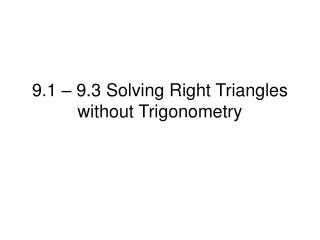DownloadDownload Presentation9.1 – 9.3 Solving Right Triangles without Trigonometry

# 9.1 – 9.3 Solving Right Triangles without Trigonometry

Download Presentation## 9.1 – 9.3 Solving Right Triangles without Trigonometry

- - - - - - - - - - - - - - - - - - - - - - - - - - - E N D - - - - - - - - - - - - - - - - - - - - - - - - - - -
##### Presentation Transcript

1. 9.1 – 9.3 Solving Right Triangles without Trigonometry

2. x 15 8 10 2. Find the length of the altitude drawn to the hypotenuse. • x = 10.95 • x = 11.5 • x = 13.56 • None of the above <Explanation follows.>

3. 8 x 23 15 x x 15 x x 8 15 8 A A C B C C D B D 2. Solution You can redraw the triangles: So, x2 = 8*15 x2 = 120 x = 10.95 (A)

4. 9 3. Find the value of x. • x = 219 • x = 437 • x = 223 • x = 23 x 19 4 <Explanation follows.>

5. 19 x x 23 A A C B C C D B D 3. Solution You can redraw the triangles: x 19 4 x 23 19 x So, x2 = 19*23 x2 = 437 x = 437 4 19

6. 10 4. The geometric mean of 5 and 15 is: • 3 • 10 • 5 3 • 75 <Explanation follows.>

7. 5 x x 15 4. Solution Geometric mean: So, x2 = 75 x = 75 x = 253 x = 53

8. 9 9. Find the value of x. • 8.3 • 7.0 • 10.1 • 1.9

9. 7 10. Choose the sets that are possible side lengths of a right triangle. • 1,1,2 • 1,1,2 • 3,4,7 • 3,4,5 • a and c • b and d

10. 10 9 10.A. Find the side length of a square with a diagonal of length 10. • 3 • 4.5 • 7.07 • 9 <Explanation follows.>

11. 10 10.A. Solution x x So, x2 + x2= 100 2x2 = 100 x2 = 50 x = 7.07

12. 11.6 6 h 10 9 15. Find the value of h. • h = 3.5 • h = 5.1 • h = 7.5 • h = 10.77 <Explanation follows.>

13. 11.6 11.6 6 10 6 10 11.6 h h 6 h 10 A A C B C C D B D 15. Solution You can redraw the triangles: 10 11.6 10 6 h h 6

14. 10 11. Which statements are true about options B and C in #11? • B is acute and C is obtuse • B is obtuse and C is acute • B is acute and C is not a triangle • B is obtuse and C is not a triangle <Explanation follows.>

15. 11. Solution • 6,7,12 • 20.8, 39, 74.2 • Put the largest first and confirm it can be a triangle. • If yes, square them and compare largest to other two: • B. 122 _____ 62 + 72 • ______ 36 + 49 • C. 74.2______20.8 + 39

16. 9 13. Find the value of x. • x = 257 • x =  133 • x = 19 • x = 221 x 12 7